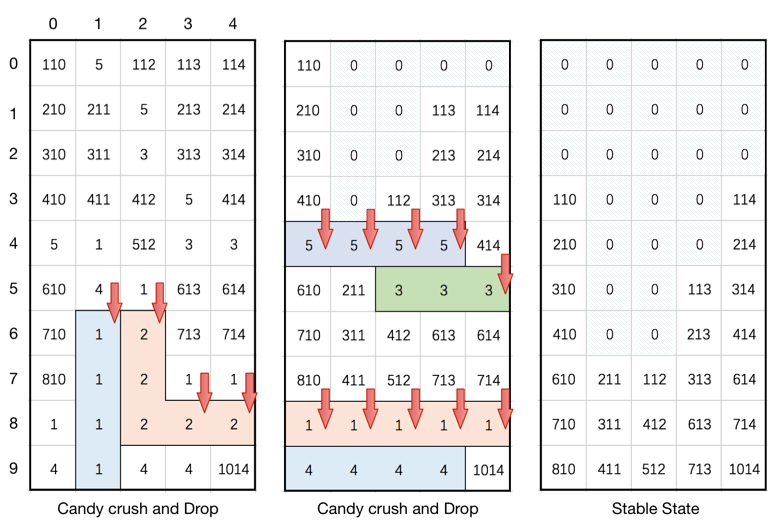# 723-candy-crush

## Question

https://leetcode.com/problems/candy-crush/description/

This question is about implementing a basic elimination algorithm for Candy Crush.

Given a 2D integer array board representing the grid of candy, different positive integers board[i][j] represent different types of candies. A value of board[i][j] = 0 represents that the cell at position (i, j) is empty. The given board represents the state of the game following the player's move. Now, you need to restore the board to a stable state by crushing candies according to the following rules:

1. If three or more candies of the same type are adjacent vertically or horizontally, "crush" them all at the same time - these positions become empty.
2. After crushing all candies simultaneously, if an empty space on the board has candies on top of itself, then these candies will drop until they hit a candy or bottom at the same time. (No new candies will drop outside the top boundary.)
3. After the above steps, there may exist more candies that can be crushed. If so, you need to repeat the above steps.
4. If there does not exist more candies that can be crushed (ie. the board is stable), then return the current board.

You need to perform the above rules until the board becomes stable, then return the current board.

Example:

``````Input:
board =
[[110,5,112,113,114],[210,211,5,213,214],[310,311,3,313,314],[410,411,412,5,414],[5,1,512,3,3],[610,4,1,613,614],[710,1,2,713,714],[810,1,2,1,1],[1,1,2,2,2],[4,1,4,4,1014]]
Output:
[[0,0,0,0,0],[0,0,0,0,0],[0,0,0,0,0],[110,0,0,0,114],[210,0,0,0,214],[310,0,0,113,314],[410,0,0,213,414],[610,211,112,313,614],[710,311,412,613,714],[810,411,512,713,1014]]
Explanation:
``````[Image taken from LeetCode]

Note:

1. The length of board will be in the range [3, 50].
2. The length of board[i] will be in the range [3, 50].
3. Each board[i][j] will initially start as an integer in the range [1, 2000].

## Thought Process

1. Pointers
1. Mark the crushed candidate directly on the board by negating the value
2. We check in group of 3, if they are the same absolute value, we mark them for crushing step
3. Time complexity O((rc)^2), because it costs 3rc to scan the whole board then we call at most rc/3 times
4. Space complexity O(1)

## Solution

``````class Solution {
public int[][] candyCrush(int[][] board) {
int r = board.length, c = board.length;
boolean crush = false;
for (int i = 0; i < r; i++) {
for (int j = 0; j < c; j++) {
// check horizontal line
int v = Math.abs(board[i][j]);
if (v == 0) continue;
if (j + 2 < c && v == Math.abs(board[i][j + 1]) && v == Math.abs(board[i][j + 2])) {
crush = true;
board[i][j] = board[i][j + 1] = board[i][j + 2] = -v;
}
// check vertical line
if (i + 2 < r && v == Math.abs(board[i + 1][j]) && v == Math.abs(board[i + 2][j])) {
crush = true;
board[i][j] = board[i + 1][j] = board[i + 2][j] = -v;
}
}
}
// crush candy
for (int j = 0; j < c; j++) {
int id = r - 1;
for (int i = r - 1; i >= 0; i--) {
if (board[i][j] > 0) {
board[id--][j] = board[i][j];
}
}
while (id >= 0) board[id--][j] = 0;
}
return crush ? candyCrush(board) : board;
}
}
``````